# Lyapunov theorem

(diff) ← Older revision | Latest revision (diff) | Newer revision → (diff)

Lyapunov's theorem in probability theory is a theorem that establishes very general sufficient conditions for the convergence of the distributions of sums of independent random variables to the normal distribution. The precise statement of Lyapunov's theorem is as follows: Suppose that the independent random variables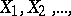have finite means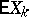, variances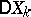and absolute moments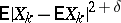,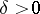, and suppose also that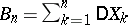is the variance of the sum of. Then if for some,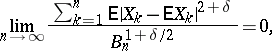(1)

the probability of the inequality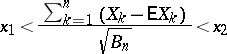(2)

tends to the limit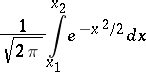(3)

as, uniformly with respect to all values of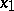and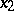. Condition (1) is called the Lyapunov condition. Lyapunov's theorem was stated and proved by A.M. Lyapunov in 1901 and was the final step in research of P.L. Chebyshev, A.A. Markov and Lyapunov on conditions for the applicability of the central limit theorem of probability theory. Later, conditions were established that extend Lyapunov's conditions and that are not only sufficient but also necessary. A final solution of the question in this direction was obtained by S.N. Bernstein [S.N. Bernshtein], J.W. Lindeberg and W. Feller. The power of the method of characteristic functions was demonstrated for the first time in Lyapunov's theorem.

Lyapunov also gave an upper bound (for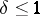) for the absolute value of the differencebetween the probability of (2) and its approximate value (3). This bound can be expressed in the following form: For,and for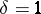,whereand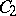are absolute constants andis the fraction (the Lyapunov fraction) under the limit sign in (1). See also Berry–Esseen inequality.

## Contents

How to Cite This Entry:
Lyapunov theorem. Encyclopedia of Mathematics. URL: http://encyclopediaofmath.org/index.php?title=Lyapunov_theorem&oldid=18024
This article was adapted from an original article by A.V. Prokhorov (originator), which appeared in Encyclopedia of Mathematics - ISBN 1402006098. See original article